SPSS TUTORIALS BASICS ANOVA REGRESSION FACTOR CORRELATION

# Independent Samples T-Test – Quick Introduction

## Independent Samples T-Test - What Is It?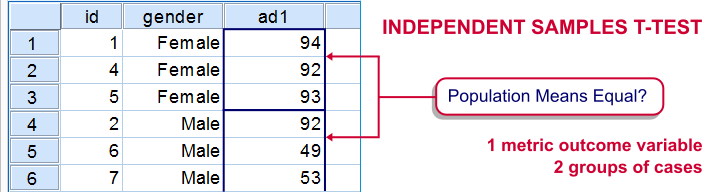An independent samples t-test evaluates if 2 populations have equal means on some variable. If the population means are really equal, then the sample means will probably differ a little bit but not too much. Very different sample means are highly unlikely if the population means are equal. This sample outcome thus suggest that the population means weren't equal after all.
The samples are independent because they don't overlap; none of the observations belongs to both samples simultaneously. A textbook example is male versus female respondents.

## Example

Some island has 1,000 male and 1,000 female inhabitants. An investigator wants to know if males spend more or fewer minutes on the phone each month. Ideally, he'd ask all 2,000 inhabitants but this takes too much time. So he samples 10 males and 10 females and asks them. Part of the data are shown below.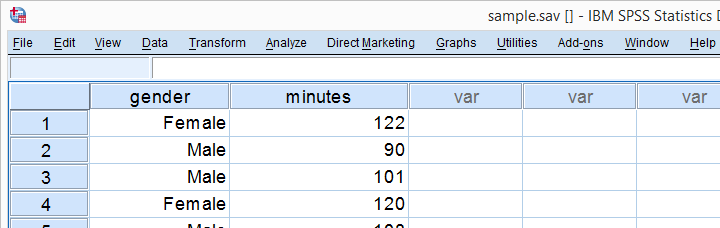Next, he computes the means and standard deviations of monthly phone minutes for male and female respondents separately. The results are shown below.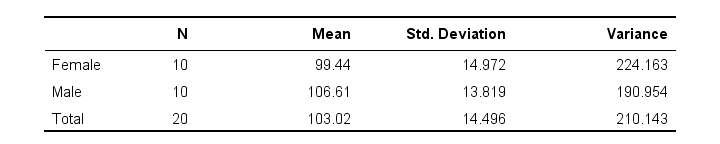These sample means differ by some (99 - 106 =) -7 minutes: on average, females spend some 7 minutes less on the phone than males. But that's just our tiny samples. What can we say about the entire populations? We'll find out by starting off with the null hypothesis.

## Null Hypothesis

The null hypothesis for an independent samples t-test is (usually) that the 2 population means are equal. If this is really true, then we may easily find slightly different means in our samples. So precisely what difference can we expect? An intuitive way for finding out is a simple simulation.

## Simulation

I created a fake dataset containing the entire populations of 1,000 males and 1,000 females. On average, both groups spend 103 minutes on the phone with a standard-deviation of 14.5. Note that the null hypothesis of equal means is clearly true for these populations.
I then sampled 10 males and 10 females and computed the mean difference. And then I repeated that process 999 times, resulting in the 1,000 sample mean differences shown below.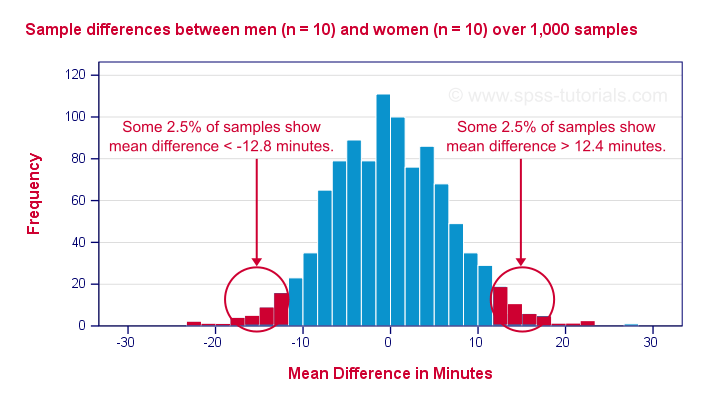First off, the mean differences are roughly normally distributed. Most of the differences are close to zero -not surprising because the population difference is zero. But what's really interesting is that mean differences between, say, -12.5 and 12.5 are pretty common and make up 95% of my 1,000 outcomes. This suggests that an absolute difference of 12.5 minutes is needed for statistical significance at α = 0.05.
Last, the standard deviation of our 1,000 mean differences -the standard error- is 6.4. Note that some 95% of all outcomes lie between -2 and +2 standard errors of our (zero) mean. This is one of the best known rules of thumb regarding the normal distribution.
Now, an easier -though less visual- way to draw these conclusions is using a couple of simple formulas.

## Test Statistic

Again: what is a “normal” sample mean difference if the population difference is zero? First off, this depends on the population standard deviation of our outcome variable. We don't usually know it but we can estimate it with $$Sw = \sqrt{\frac{(n_1 - 1)\;S^2_1 + (n_2 - 1)\;S^2_2}{n_1 + n_2 - 2}}$$
in which $$Sw$$ denotes our estimated population standard deviation. For our data, this boils down to $$Sw = \sqrt{\frac{(10 - 1)\;224 + (10 - 1)\;191}{10 + 10 - 2}} ≈ 14.4$$
Second, our mean difference should fluctuate less -that is, have a smaller standard error- insofar as our sample sizes are larger. The standard error is calculated as $$Se = Sw\sqrt{\frac{1}{n_1} + \frac{1}{n_2}}$$
and this gives us $$Se = 14.4\; \sqrt{\frac{1}{10} + \frac{1}{10}} ≈ 6.4$$
If the population mean difference is zero, then -on average- the sample mean difference will be zero as well. However, it will have a standard deviation of 6.4. We can now just compute a z-score for the sample mean difference but -for some reason- it's called T instead of Z: $$T = \frac{\overline{X}_1 - \overline{X}_2}{Se}$$
which, for our data, results in $$T = \frac{99.4 - 106.6}{6.4} ≈ -1.11$$
Right, now this is our test statistic: a number that summarizes our sample outcome with regard to the null hypothesis. T is basically the standardized sample mean difference; T = -1.11 means that our difference of -7 minutes is roughly 1 standard deviation below the average of zero.

## Assumptions

Our t-value follows a t distribution but only if the following assumptions are met:

1. Independent observations or, precisely, independent and identically distributed variables.
2. Normality: the outcome variable follows a normal distribution in the population. This assumption is not needed for reasonable sample sizes (say, N > 25).
3. Homogeneity: the outcome variable has equal standard deviations in our 2 (sub)populations. This is not needed if the sample sizes are roughly equal. Levene's test is sometimes used for testing this assumption.

If our data meet these assumptions, then T follows a t-distribution with (n1 + n2 -2) degrees of freedom (df). In our example, df = (10 + 10 - 2) = 18. The figure below shows the exact distribution. Note that we need an absolute t-value of 2.1 for 2-tailed significance at α = 0.05.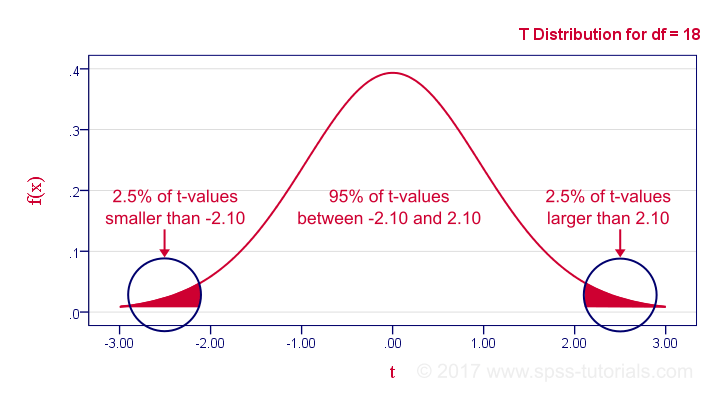Minor note: as df becomes larger, the t-distribution approximates a standard normal distribution. The difference is hardly noticeable if df > 15 or so.

## Statistical Significance

Last but not least, our mean difference of -7 minutes is not statistically significant: t(18) = -1.11, p ≈ 0.28. This means we've a 28% chance of finding our sample mean difference -or a more extreme one- if our population means are really equal; it's a normal outcome that doesn't contradict our null hypothesis.
Our final figure shows these results as obtained from SPSS.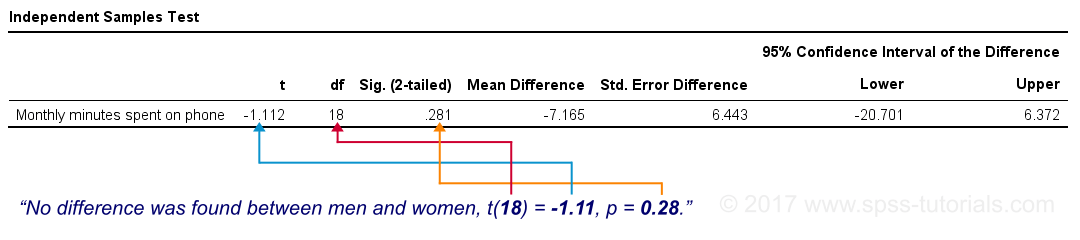## Effect Size

Finally, the effect size measure that's usually preferred is Cohen’s D, defined as $$D = \frac{\overline{X}_1 - \overline{X}_2}{Sw}$$

in which $$Sw$$ is the estimated population standard deviation we encountered earlier. That is, Cohen’s D is the number of standard deviations between the 2 sample means. So what is a small or large effect? The following rules of thumb have been proposed:

• D = 0.20 indicates a small effect;
• D = 0.50 indicates a medium effect;
• D = 0.80 indicates a large effect.

Cohen’s D is painfully absent from SPSS except for SPSS 27. However, you can easily obtain it from Cohens-d.xlsx. Just fill in 2 sample sizes, means and standard deviations and its formulas will compute everything you need to know.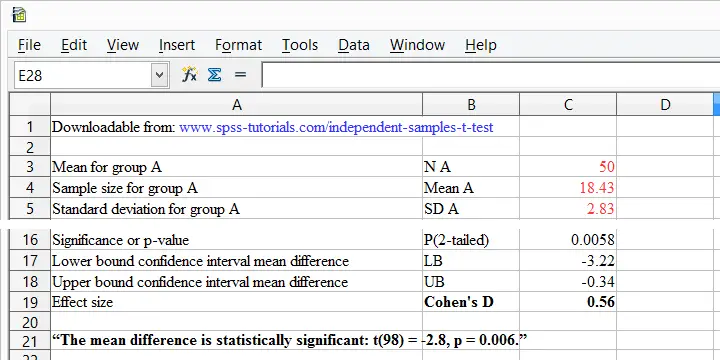# Tell us what you think!

*Required field. Your comment will show up after approval from a moderator.

# THIS TUTORIAL HAS 29 COMMENTS:

•### By Ruben Geert van den Berg on March 18th, 2019

Hi Zahid!

Sure! The appropriate test depends on their measurement levels. If both can be considered metric, then a t-test is probably the way to go. For a more complete overview of the options, please see Simple Overview Statistical Comparison Tests.

Hope that helps!

•### By Abdulkerim on July 29th, 2019

Thank you for your nice description.
I want to know how to know the correlation between metric and nominal data if the nominal data has more than two categories and the metric data is the independent data.
For example if I want to check whether the lesion size determines the complication outcome during procedures. The lesion size is metric data (in cm) which is also the independent variable and the complication is a nominal data which is dependent variable and has multiple choices.

•### By Ruben Geert van den Berg on July 29th, 2019

Hi Abdulkerim!

Good question!

The basic analysis for this is ANOVA.

The usual effect size measure for this is partial eta squared.

It's comparable to a squared correlation in that it's the proportion of variance accounted for. However, it's not literally a correlation.

I suggest you report an ANOVA as usual -with statistical significance- and add eta squared to the table.

Hope that helps!

SPSS tutorials

•### By Cynthia Terrell on June 13th, 2020

Very good tutorial, just a lot of information. I will have to revisit this a lot I know. Brings support to me for my research project. Thanks

•### By Harrietta Akrofi-Ansah on August 6th, 2020

The topics are great and mind blowing!